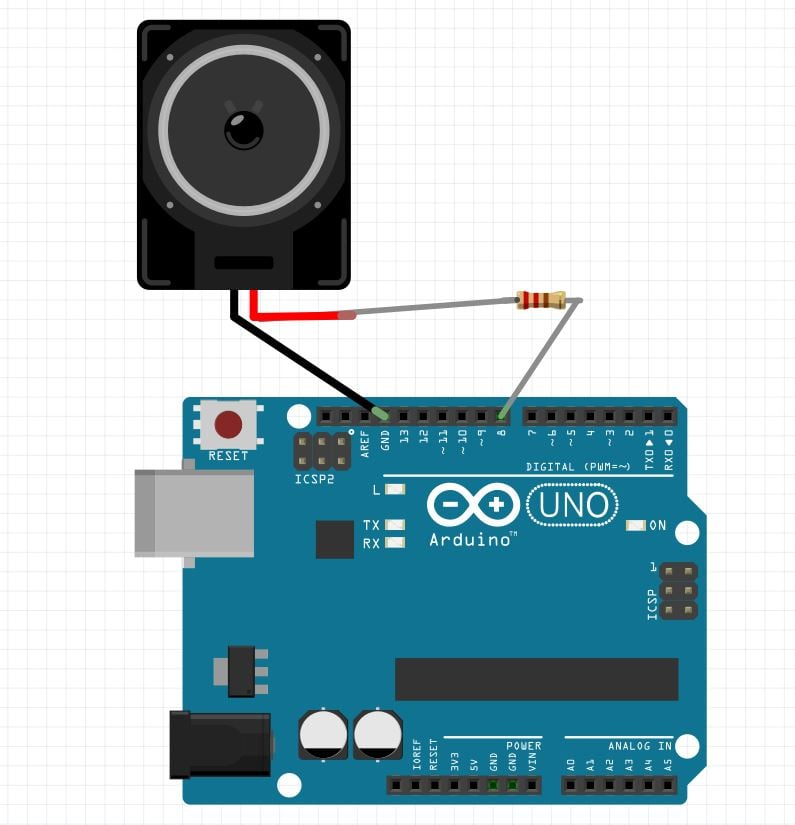Beauty of mathematical music!

New journey has begun where ideas can be easily implementedusing open source community (thanks to Arduino). So here is a way:

· Look around yourself andobserve your surrounding

· Discover problems that need to be solved

· Think, try, and keep learning and share

· Most important, what I call is KEEP IT SIMPLE :-)

· Repeat

My intention was to make a musical-based project using Arduino platform.

So I searched over internet and found amazing creative projects but I wanted to create my own stuff!

So I came across this tone() function from arduino library.Below is a link you can find descriptive information about it

Basically tone() function as you would have read generates sound of some frequency you pass it in a parameter. So I thought how can I use this single function to generate different kinds of sound?

The answer was in mathematics. We all use math everyday. It's logic is about solving mysteries of this universe (sentence from famous Numb3rs TV series)...

The idea is to make sound which can be generated using mathematical equations. Let’s call it "MathMusic."

In this project, I used:

· Area’s equation of simple geometrical shapes (square, rectangle, parallelogram, triangle, and circle)

· Quadratic equation and Fibonacci seres is

To generate sound. Now you can expand this project and discover some new music using different equations or formula…

## SchematicSchematic